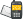It is currently 28 Nov 2020, 14:47### GMAT Club Daily Prep

#### Thank you for using the timer - this advanced tool can estimate your performance and suggest more practice questions. We have subscribed you to Daily Prep Questions via email.

Customized
for You

we will pick new questions that match your level based on your Timer History

Track

every week, we’ll send you an estimated GMAT score based on your performance

Practice
Pays

we will pick new questions that match your level based on your Timer History

#### Not interested in getting valuable practice questions and articles delivered to your email? No problem, unsubscribe here.# The sequence a1, a2, a3, …, an is defined by an = 9 + an – 1Question banks Downloads My Bookmarks Reviews Important topics
Author Message
TAGS:
Retired ModeratorJoined: 07 Jun 2014
Posts: 4803
GRE 1: Q167 V156WE: Business Development (Energy and Utilities)
Followers: 175

Kudos [?]: 3036 , given: 394

The sequence a1, a2, a3, …, an is defined by an = 9 + an – 1 [#permalink]
Expert's post00:00

Question Stats:83% (01:11) correct16% (01:35) wrongbased on 42 sessions
The sequence $$a_1, a_2, a_3, ....,$$ an is defined by $$a_n = 9 + a_{n – 1}$$ for each integer n ≥ 2. If $$a_1 = 11$$, what is the value of $$a_{35}$$?

[Reveal] Spoiler: OA
317

_________________

Sandy
If you found this post useful, please let me know by pressing the Kudos Button

Try our free Online GRE TestModeratorJoined: 07 Jan 2018
Posts: 697
Followers: 11

Kudos [?]: 785  , given: 88

Re: The sequence a1, a2, a3, …, an is defined by an = 9 + an – 1 [#permalink]
1
KUDOS
We are looking to find the value of 35th integer.
We know the value of first term=11
There remains 34 more. Each term after 1st increases by 9 hence the 35th term is given by 11+ 34*9 = 317

Posted from my mobile device_________________

Need Practice? 20 Free GRE Quant Tests available for free with 20 Kudos

Retired ModeratorJoined: 07 Jun 2014
Posts: 4803
GRE 1: Q167 V156WE: Business Development (Energy and Utilities)
Followers: 175

Kudos [?]: 3036 , given: 394

Re: The sequence a1, a2, a3, …, an is defined by an = 9 + an – 1 [#permalink]
Expert's post
Explanation

Each term in the sequence is 9 greater than the previous term. To make this clear, write a few terms of the sequence: 11, 20, 29, 38, etc.

$$a_{35}$$ comes 34 terms after $$a_1$$ in the sequence. In other words, $$a_{35}$$ is $$34 \times 9 = 306$$ greater than $$a_1$$.

Thus, $$a_{35} = 11 + 306 = 317$$.
_________________

Sandy
If you found this post useful, please let me know by pressing the Kudos Button

Try our free Online GRE Test

InternJoined: 06 Jul 2018
Posts: 32
Followers: 0

Kudos [?]: 3 , given: 3

Re: The sequence a1, a2, a3, …, an is defined by an = 9 + an – 1 [#permalink]
34×9+11=317
Senior ManagerJoined: 10 Feb 2020
Posts: 496
Followers: 2

Kudos [?]: 137 , given: 300

Re: The sequence a1, a2, a3, …, an is defined by an = 9 + an – 1 [#permalink]
sandy wrote:
Explanation

Each term in the sequence is 9 greater than the previous term. To make this clear, write a few terms of the sequence: 11, 20, 29, 38, etc.

$$a_{35}$$ comes 34 terms after $$a_1$$ in the sequence. In other words, $$a_{35}$$ is $$34 \times 9 = 306$$ greater than $$a_1$$.

Thus, $$a_{35} = 11 + 306 = 317$$.

_________________

Ever Tried? Ever Failed? No Matter. Try Again. Fail Again. Fail Better!!ManagerJoined: 09 Mar 2020
Posts: 164
Followers: 0

Kudos [?]: 150  , given: 16

Re: The sequence a1, a2, a3, …, an is defined by an = 9 + an – 1 [#permalink]
1
KUDOS
Farina wrote:
sandy wrote:
Explanation

Each term in the sequence is 9 greater than the previous term. To make this clear, write a few terms of the sequence: 11, 20, 29, 38, etc.

$$a_{35}$$ comes 34 terms after $$a_1$$ in the sequence. In other words, $$a_{35}$$ is $$34 \times 9 = 306$$ greater than $$a_1$$.

Thus, $$a_{35} = 11 + 306 = 317$$.

The formula is Tn = a + (n-1)d, a = first term, n = term to find, d = difference between two consecutive terms (since 9 is added with every term, that is the difference)
Where T35 = 11 + (35-1) 9
T35 = 11 + (34) 9
T35 = 317Re: The sequence a1, a2, a3, …, an is defined by an = 9 + an – 1   [#permalink] 23 May 2020, 23:22
Display posts from previous: Sort by

# The sequence a1, a2, a3, …, an is defined by an = 9 + an – 1Question banks Downloads My Bookmarks Reviews Important topicsPowered by phpBB © phpBB Group Kindly note that the GRE® test is a registered trademark of the Educational Testing Service®, and this site has neither been reviewed nor endorsed by ETS®.